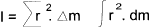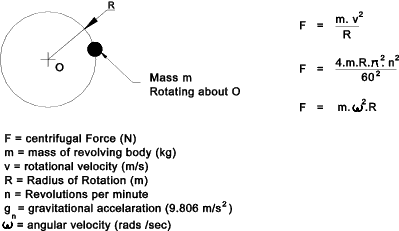Formulae_Index
Nomenclature

Dynamics Formulae

 Linear Motion For constant velocity. Distance moved at constant velocity s = v . t For constant Acceleration from velocity v_o start. v = v_o + a . t s = v_o . t + 1/2 . a . t2 v2 = v_o2 + 2 . a . s Simple Harmonic Motion Simple Harmonic Motion is characterised by the relationship that the rate of acceleration of a body towards a central position is directly related to the distance of the body from the central position. The result is a cyclic motion with a frequency (Cycles/s) = ω / 2 . p   Cycles/s.   Note: ω = angular velocity in rads/s f ''(x) = - ω2 . x The solution for the displacement from and the velocity towards the central position is below; x = a . cos ( ω. t + ε ) x ' = - ω . a . sin ( ω . t + ε ) Newtons laws of Motion Newtons First Law; Every body continues in a state of rest or of uniform rectilinear motion unless acted upon by a force. Newtons Second law; The time rate of change of linear momentum of a body is proportional to the unbalanced force acting on the body and occurs in the direction in which the force acts. Newtons Third law To each action (or force) there is an equal and opposite reaction. The mutual force of the two bodies acting upon each other are equal in magnitude, opposite in direction and are colinear. Momentum Momentum = m . v Force F = m . a Mass Moment of Inertia The mass moment of inertia of a body about an axis has been defined as the sum of the products of mass-elements and the square of their distance from the axisMass Moment Of Inertia of Various Solids Rotary Motion For a mass rotating about a centre. The force tending to accelarate the mass towards the centre and restraining the mass to move around the centre is the centripetral force. The reaction to this force tending the accelarate the mass away is the centrifugal force. (If the string breaks the mass would fly away under the effect of the centrifugal force.Acceleration Torque Angular acceleration  α = (ω2 - ω1) / t Acceleration Torque   T = I . α

Formulae_Index
Nomenclature